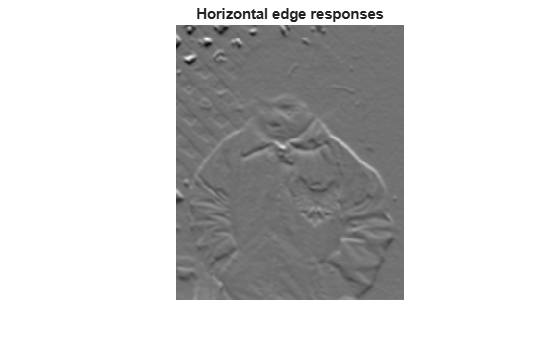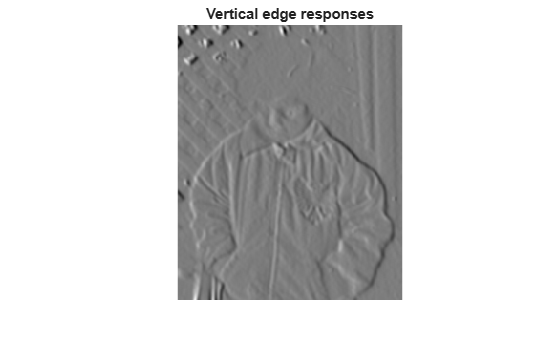Documentation

This is machine translation

Mouseover text to see original. Click the button below to return to the English version of the page.

Find Vertical and Horizontal Edges in Image

Construct Haar-like wavelet filters to find vertical and horizontal edges in an image.

Read the input image and compute the integral image.

```I = imread('pout.tif'); intImage = integralImage(I);```

Construct Haar-like wavelet filters. Use the dot notation to find the vertical filter from the horizontal filter.

```horiH = integralKernel([1 1 4 3; 1 4 4 3],[-1, 1]); vertH = horiH.'```
```vertH = integralKernel with properties: BoundingBoxes: [2x4 double] Weights: [-1 1] Coefficients: [4x6 double] Center: [2 3] Size: [4 6] Orientation: 'upright' ```

Display the horizontal filter.

`imtool(horiH.Coefficients, 'InitialMagnification','fit');`Compute the filter responses.

```horiResponse = integralFilter(intImage,horiH); vertResponse = integralFilter(intImage,vertH);```

Display the results.

```figure; imshow(horiResponse,[]); title('Horizontal edge responses');``````figure; imshow(vertResponse,[]); title('Vertical edge responses');```